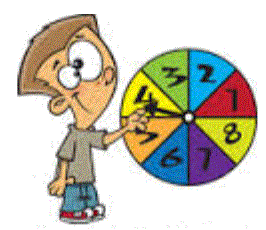# Probability Skill Test - Higher

10 Questions | Total Attempts: 47SettingsMiscellanous probability questions

• 1.
In a group of 50 students, 10 play both football and cricket, 20 play only football and 15 do not play either.  What are the values of abc and d in the Venn diagram?a = [Blank]b = [Blank]c = [Blank]d = [Blank]
• 2.
Two fair six-sided dice are rolled and the two scores are multiplied. What is the probability that the product of the scores is an even number?(Give your answer as a percentage)
• 3.
A spinner has 8 equal sections: 3 are red, 4 are blue and 1 is yellow. The spinner is spun twice.What is the probability of getting a red on the first spin and a blue on the second spin?(Give the exact answer as a percentage)
• 4.
A counter is removed from a bag and then replaced before a second counter is removed. Use the tree diagram to find the probability that two black counters are removed.(Give your answer as a percentage)
• 5.
Use the Venn diagram to calculate P(A│B).P(A│B) means P(A given B)(Give your answer as a decimal number)
• 6.
The two-way table shows the number of left-handed and right-handed boys and girls in a class.One boy and one girl are chosen at random from the class. What is the probability that both the boy and the girl are left-handed?   ......................%
• 7.
A survey is done in a junior school and it is found that 45 of the 90 boys and 33 of the 110 girls cycle to school.Find the values for abcdef in the tree diagram. Type your answers as decimals in the gaps below.a = [Blank]b = [Blank]c = [Blank]d = [Blank]e = [Blank]f = [Blank]
• 8.
The table shows the numbers of year 10 and year 11 students who attend a science club. Calculate the probabilities below and answer using percentages. P(a student chosen at random is a girl) = [Blank]%P(a boy chosen at random is in year 10) = [Blank]%
• 9.
A teacher chooses 1 boy and 1 girl from her class for an experiment. The tree diagram shows the probabilities that they are left- or right-handed. What is the probability that one of the students she picks is left-handed and the other is right-handed?(Give your answer as a decimal number)
• 10.
Set T = the first ten multiples of 2.Set F = the first ten multiples of 3.Give the set T∩F, listing the elements in ascending order: T∩F = {[Blank],[Blank],[Blank]}Back to top